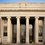# Discussion: Photon Yoga

An object has the shape of a square and has side length $a$. Light beams are shone on the object from a big machine. If $m$ is the mass of the object, $P$ is the power per unit area of the photons, $c$ is the speed of light, and $g$ is the acceleration of gravity, prove that the minimum value of $P$ such that the bar levitates due to the light beams is $P = \dfrac {4cmg}{5a^2}.$

Note: This problem was originally proposed by Trung Phan for the IPhOO.Note by Ahaan Rungta
7 years, 3 months ago

This discussion board is a place to discuss our Daily Challenges and the math and science related to those challenges. Explanations are more than just a solution — they should explain the steps and thinking strategies that you used to obtain the solution. Comments should further the discussion of math and science.

When posting on Brilliant:

• Use the emojis to react to an explanation, whether you're congratulating a job well done , or just really confused .
• Ask specific questions about the challenge or the steps in somebody's explanation. Well-posed questions can add a lot to the discussion, but posting "I don't understand!" doesn't help anyone.
• Try to contribute something new to the discussion, whether it is an extension, generalization or other idea related to the challenge.

MarkdownAppears as
*italics* or _italics_ italics
**bold** or __bold__ bold
- bulleted- list
• bulleted
• list
1. numbered2. list
1. numbered
2. list
Note: you must add a full line of space before and after lists for them to show up correctly
paragraph 1paragraph 2

paragraph 1

paragraph 2

[example link](https://brilliant.org)example link
> This is a quote
This is a quote
    # I indented these lines
# 4 spaces, and now they show
# up as a code block.

print "hello world"
# I indented these lines
# 4 spaces, and now they show
# up as a code block.

print "hello world"
MathAppears as
Remember to wrap math in $$ ... $$ or $ ... $ to ensure proper formatting.
2 \times 3 $2 \times 3$
2^{34} $2^{34}$
a_{i-1} $a_{i-1}$
\frac{2}{3} $\frac{2}{3}$
\sqrt{2} $\sqrt{2}$
\sum_{i=1}^3 $\sum_{i=1}^3$
\sin \theta $\sin \theta$
\boxed{123} $\boxed{123}$

Sort by:

Are the photons striking perpendicularly? Are they rebounding? (Please clarify.)

If both of above assumptions are valid, using classical mechanics, I get $P = \frac{cmg}{2a^2}$

- 7 years, 3 months ago

This is the issue. We did not mention any assumptions!

- 7 years, 3 months ago

each photon (assuming it strikes and rebounds perpendicularly) grants a momentum of 2E/c E=2Pta^2/c so we need mgt=2E/c=2Pta^2/c so P=cmg/2a^2

- 7 years, 3 months ago

Ahaan, can you suggest something Jatin and I might be missing?

Staff - 7 years, 3 months ago

We gave a statement to prove and said nothing about how the light is reflected. Some investigation should suggest that it's reflected in the way that the probability for the ray to scatter in any direction is equal.

- 7 years, 3 months ago

Hey, ahaan, even if we assume that the incidence and scattering is random, we get $P = \dfrac{\pi cmg}{4 a^2}$, not $\dfrac{4cmg}{5a^2}$

Initial vertical momentum/sec = $\dfrac{Pa^2}{c} \displaystyle \int_{- \frac{\pi}{2}}^{\frac{\pi}{2}} \cos \theta \frac{d \theta}{\pi} = \dfrac{2 P a^2}{\pi c}$.

Final vertical momentum/sec = $- \dfrac{2P a^2}{\pi c}$

Hence, Force = $\dfrac{4P a^2}{\pi c}$

Hence, $P_{min} = \dfrac{\pi cmg }{4 a^2}$, which is quite close to the answer you show, but not exactly equal!

- 7 years, 3 months ago

@jatin yadav and all others, sorry to bother you here. How much are you expecting in mains Jatin?

- 7 years, 3 months ago

You did this problem in two dimensions. It is a three-dimensional problem. You need to take into account a trigonometric factor, resulting in a different integral. If you want, I can tell you the correct integral but I'd prefer not to as I want everybody to discover the correct solution themselves!

- 7 years, 3 months ago

we ... said nothing about how the light is reflected

Right, so, given that it's unspecified we are free to think of whatever arrangement for the geometry of the light shining onto the surface, right?

Light shining perpendicularly onto the square results in the most efficient momentum transfer to the square.

Like Jatin said, under that assumption (that the light shines perpendicularly), it is possible the levitate the bar with $P = \frac{cmg}{2a^2}$ which is less than $\frac{4cmg}{5a^2}$, contradicting the claim in the problem that $P = \frac{cmg}{2a^2}$ is the minimum power that can achieve levitation.

The only possibilities are that Jatin and I are reading the problem wrong, or there is something wrong with our argument, or possibly that the claim is wrong. Can you perhaps point out where we might be going wrong in our reading of the problem?

Staff - 7 years, 3 months ago

Right, so, given that it's unspecified we are free to think of whatever arrangement for the geometry of the light shining onto the surface, right?

Which is why we scored everybody who got a $\frac {1}{2}$-coefficient 5/7. I will come up with a better reasoning soon.

- 7 years, 3 months ago